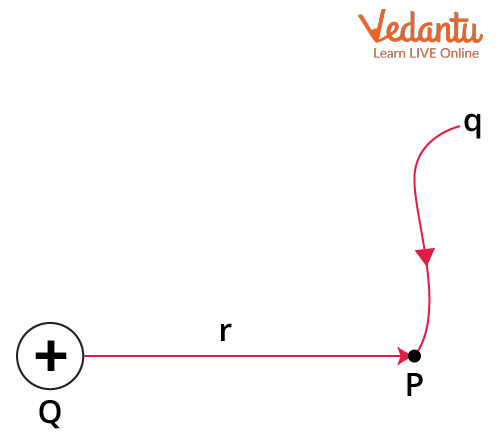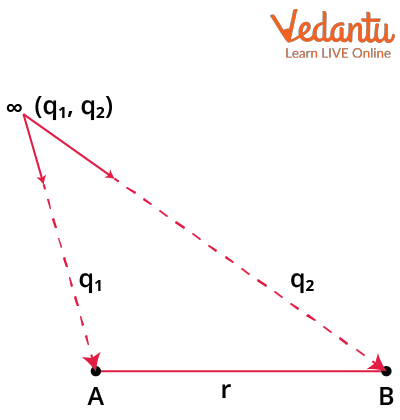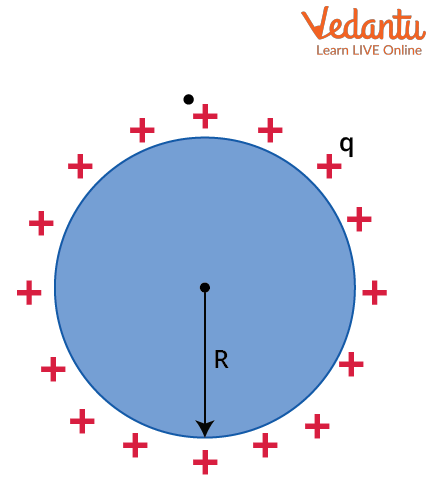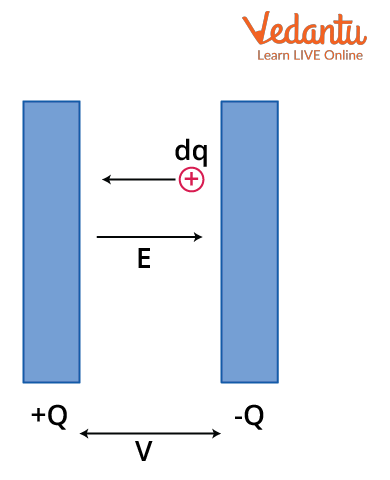Courses
Courses for Kids
Free study material
Free LIVE classes
More

# Electrostatic Potential and Capacitance for JEELIVE
Join Vedantu’s FREE Mastercalss

## Introduction to Electrostatic Force

Electric or electrostatic force was discovered by Charles Coulomb, who tried to measure the force between two charged objects with the help of a torsion balance. Coulomb’s law is similar to Newton's law of gravitation. Coulomb's law states that the electrostatic force between any two charged particles is directly proportional to the product of their charges and inversely proportional to the square of the distance between them. This force is along the line joining the two charges, and it can be represented as follows,

$F=\dfrac{1}{4 \pi \varepsilon_{0}} \dfrac{q_{1} q_{2}}{r^{2}}$

Where q1 and q2 are the two charged particles, r is the distance between them and

$\dfrac{1}{4 \pi \varepsilon_{\mathrm{o}}}$ coulomb’s constant, whose value is 9 $\times$ 109 Nm2C-2. This force becomes attractive when the two charges have opposite signs. Similarly, it becomes repulsive if they have the same sign. Let us learn how the electrostatic potential is defined and derived using the electrostatic force equation in this article.

## Electrostatic Potential and Energy

### Electrostatic Potential

Electrostatic potential at any point P is defined as the negative of the work done by the electrostatic field to bring a unit positive charge from infinity to the point of observation P. It is denoted by V and is measured in volts. This is simply described as the work done per unit positive charge and represented as

$V=\dfrac{W}{q}$

Where q is the unit positive charge and W is the work done.

Let us derive the equation for potential due to a point charge in an electrostatic field. Start by assuming that a point charge Q is placed in a static electric field and a point P is considered at a distance r as shown in the figure.Electrostatic potential at point P

Electrostatic potential at point P is the work done to bring unit charge from infinity to point P. Let us find the work done by slowly moving the unit charge from infinity to point P. Let us assume the unit positive charge q is at point P and the force acting at point P due to the charge Q is given by the equation,

$F=\dfrac{1}{4 \pi \varepsilon_{0}} \dfrac{Q q}{r^{2}}$ - - - -  - (1)

Where q is the unit positive charge and Q is the point charge assumed in the electrostatic field.

Work done by the electrostatic field to move the charge from infinity distance to a distance of r  is given by,

$\mathrm{W}=-\int_{\infty}^{r} F \cdot d r$

Substituting the value of (1) in the above equation, we obtain

\begin{align} &W=-\int_{\infty}^{r} \dfrac{1}{4 \pi \varepsilon_{0}} \dfrac{Q q}{r^{2}} \cdot d r=\dfrac{-Q q}{4 \pi \varepsilon_{0}} \int_{\infty}^{r} \dfrac{1}{r^{2}} \cdot d r \\ &W=\dfrac{-Q q}{4 \pi \varepsilon_{0}}\left[-\dfrac{1}{r}\right]_{\infty}^{r} \\ &W=\dfrac{Q q}{4 \pi \varepsilon_{0}}\left[\dfrac{1}{r}\right] \\ &W=\dfrac{1}{4 \pi \varepsilon_{0}} \cdot \dfrac{Q q}{r}- - - - - - (2) \end{align}

This is the work done to move the charge q from infinity to r distance. Now the electrostatic potential is the work done to bring the unit positive charge to bring from infinity to P and this is represented as

$V=\dfrac{W}{q}$

Putting the value of (2) in the above equation, we obtain

$\mathrm{V}=\dfrac{W}{q}=\dfrac{\dfrac{1}{4 \pi \varepsilon_{0}} \cdot \dfrac{Q q}{r}}{q}=\dfrac{1}{4 \pi \varepsilon_{0}} \cdot \dfrac{Q}{r}$

This is the derived equation for the electrostatic potential due to a point charge. Now let us see what electrostatic potential energy.

## Electrostatic Potential Energy

Let us assume there are two charges q1 and q2 which are at infinity as shown in the figure below. Let us find the work done to bring these charges to points A and B.Electrostatic potential energy of two charges

Initially, there is no presence of an electric field so the work done to bring q1 from infinity to the point A, i.e., W1 = 0

Now, the presence of q1 at A brings a potential at B and that is represented as VB

$\mathrm{V}_{\mathrm{B}}=\dfrac{1}{4 \pi \varepsilon_{\mathrm{o}}} \cdot \dfrac{q_{1}}{r}$

Work done to bring q2 from infinity to B is W2 = q2VB

I.e.,$\mathrm{W}_{2}=\mathrm{q}_{2} \cdot\left(\dfrac{1}{4 \pi \varepsilon_{\mathrm{o}}} \cdot \dfrac{q_{1}}{r}\right)$

Therefore, $\mathrm{W}_{2}=\dfrac{1}{4 \pi \varepsilon_{\mathrm{o}}} \cdot \dfrac{q_{1} q_{2}}{r}$

The total work done in bringing both q1 and q2 from infinity to A and B is

$\mathrm{W}=\mathrm{W}_{1}+\mathrm{W}_{2}=0+\dfrac{1}{4 \pi \varepsilon_{0}} \cdot \dfrac{q_{1} q_{2}}{r}=\dfrac{1}{4 \pi \varepsilon_{0}} \cdot \dfrac{q_{1} q_{2}}{r}=\mathrm{U}$

This is the total work done to bring both charges q1 and q2 from infinity to points A and B, which becomes the potential energy of the system containing two charges. The potential energy is denoted by U and the units are in joules. Now, let us find how we relate this with capacitance and know more about the electrostatic potential and capacitance formulas, including electrostatic potential and capacitance derivations.

## Capacitance

### The Capacitance of a Spherical Conductor:

Let us take an isolated spherical conductor with a charge of q spread over it and the radius of the sphere is R as shown in the figure.Capacitance of a spherical conductor

The potential of the sphere is given by,

$\mathrm{V}=\dfrac{1}{4 \pi \varepsilon_{0}} \cdot \dfrac{Q}{R}$

Here, the potential of an isolated sphere is directly proportional to the charge provided,

I.e., $V \propto Q$

The ratio of charge provided and the potential rise in a capacitor is constant, which is defined as the capacitance.

I.e., $C=\dfrac{Q}{V}$

Here, if V = 1, then C = Q. So, this is also defined as the charge required to raise the potential of the conductor by 1 volt. The unit of capacitance is in Farads which is equal to coulomb per volt. In general, the capacitance of a capacitor is minimal in microfarads.

We know that the potential of a spherical capacitor is,

$\mathrm{V}=\dfrac{1}{4 \pi \varepsilon_{\mathrm{o}}} \cdot \dfrac{Q}{R}$

We know that, $C=\dfrac{Q}{V}=\dfrac{Q}{\dfrac{1}{4 \pi \varepsilon_{0}} \cdot \dfrac{Q}{R}}=4 \pi \varepsilon_{0} \mathrm{R}$

This is the capacitance of a spherical conductor.

## Energy Stored in a Capacitor:

The capacitor is represented as two parallel plates with the same but opposite charges separated by the distance, as shown in the figure. The charge is moved from the positive plate to the negative plate.Energy stored in a parallel plate capacitor

The capacitor is connected to a battery and the electric field starts flowing from the positive plate to the negative plate. Let us assume a charge of +dq is moving from the negative plate to the positive plate against the electric field. The work done by the battery will be

dW = Vdq

Where we know, Q = CV

$dW = \dfrac{Q}{C} \cdot \mathrm{dq}$

Integrating on both sides to find the work done by the battery for sending the charge Q from negative plate to positive plate,

$\int d W=\int_{0}^{Q} \dfrac{q}{C} \cdot d q=\dfrac{Q^{2}}{2 C}$

This is the work done by the battery, which is stored in the capacitor in the form of electrostatic field energy and is denoted by U. Therefore, we learned electrostatic potential and capacitance derivations, and now let us solve some problems.

## Solved Examples

Example 1: Find the potential at a point S by the charge q = 2.5 $\times$ 10-8 C  which is at a distance of 0.5meters from the charge q.

Solution:

Given that, q = 2.5 $\times$ 10-8 C and R = 0.5m

We know that potential at point S by the charge q is given by the formula,

$\mathrm{V}=\dfrac{1}{4 \pi \varepsilon_{0}} \cdot \dfrac{q}{R}$

Where the value of $\dfrac{1}{ 4 \pi \varepsilon_{0}}$ is the value of coulomb’s constant and is equal to  9 x 109 Nm2C-2.

After putting all the given values in the above formula, we obtain;

$V=9 \times 10^{9} \cdot \dfrac{2.5 \times 10^{-8}}{0.5}$

$\therefore V=450 \mathrm{~V}$

Therefore the potential at a point S due to charge q = 2.5 $\times$ 10-8 C is 450volts.

Example 2: Find the voltage across the capacitor when the energy stored in the capacitor is 4.2 $\times$ 1020J with the capacitance of 1.4 $\times$ 10-6F

Solution:

Given that, Energy stored, U =  4.2 $\times$ 1020J and C = 1.4 $\times$ 10-6

We know that energy stored in a capacitor is given by the formula,

$U=\dfrac{Q^{2}}{2 C}$

Put the values of U and C to get the value of Q,

Q2 = 2UC = 2.(4.2 $\times$ 1020).(1.4 $\times$ 10-6) = 11.76 $\times$ 1014

Q = 3.43 $\times$ 107C

Therefore, the charge between the plates of the capacitor is given by 3.43 $\times$ 107 C.

Now, we know that Q = CV

Where $V=\dfrac{Q}{C}$

Putting the values of Q and C in the above equation,

$V=\dfrac{3.43 \times 10^{7}}{1.4 \times 10^{-6}}$

Therefore, V = 2.45 $\times$ 1013

The required potential between the plates of a capacitor is V = 2.45 $\times$ 1013 Volts.

## Conclusion

The electrostatic potential and capacitance formulas are used in many concepts while finding potential or potential energy across many conductors in electrostatics. Through this article, we learnt many important derivations of electrostatic potential and capacitance. along with the electrostatic potential and capacitance solutions to problem examples.

Last updated date: 26th Sep 2023
Total views: 2.1k
Views today: 0.02k

## FAQs on Electrostatic Potential and Capacitance for JEE

1. What are the definitions of electrostatic potential and capacitance?

The electrostatic potential is defined as the work done in bringing a unit positive charge from infinity to a point of observation P. It is denoted by V and is measured in volts. Capacitance is the measure of the charge required to raise the potential between the parallel plates of a capacitor by 1 volt. It is denoted by C and measured in farads. One farad is a coulomb per unit charge. The electrostatic potential and capacitance are related by the formula, Q = CV.

2. What is the weightage of this topic in JEE?

The topics of electrostatic potential and capacitance are very important in the JEE exam. There will be a maximum of 1 to 2 questions on this topic with a weightage of 6.66% in JEE. The electrostatic potential and capacitance solutions to many problems are very important and are asked in various ways in the examination. So, learning electrostatic potential and capacitance formulas along with important derivations of electrostatic potential and capacitance are essential to scoring higher marks in examinations.